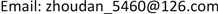﻿ 基于AHP-可拓物元公交服务质量评价 Quality Evaluation of Public Transport Service Based on AHP-Extensible Matter Element

Open Journal of Transportation Technologies
Vol. 09  No. 03 ( 2020 ), Article ID: 35707 , 10 pages
10.12677/OJTT.2020.93022

Quality Evaluation of Public Transport Service Based on AHP-Extensible Matter Element

Dan Zhou1, Panyue Qin1, Wenyong Li1,2, Hongzhuan Zhao1

1School of Architecture and Transportation Engineering, Guilin University of Electronic Technology Guilin, Guilin Guangxi

2Guangxi Transport Vocational and Technical College, Nanning GuangxiReceived: May 4th, 2020; accepted: May 15th, 2020; published: May 22nd, 2020ABSTRACT

Evaluation of public transport service quality is one of the important links to effectively improve the attractiveness of urban ground public transportation. In this paper, a bus service quality evaluation method based on AHP-extension matter element was proposed. According to the hierarchical analysis of urban road bus service quality indicators, three levels of line network planning, route layout and operation services were selected as the criterion layer; 8 main evaluation indicators were selected as the indicator layer. And the service quality evaluation indicator system was established. Using analytic hierarchy process and expert survey method, the weights of the criterion layer and the indicator layer were determined. Then, using the idea of extension matter element, the quality of public transportation service was evaluated. Finally, Guilin is taken as an example to evaluate service quality. The comprehensive evaluation level of the public transport service quality in Guilin has been third. The three criteria layers for line network planning, route layout and operation services have been second, second and third. Then, fuzzy comprehensive evaluation is used to verify the results and determine the reliability of the results. According to the evaluation results, it can be seen that the quality of public transportation services in Guilin is average and needs further improvement. And according to the evaluation level of each criterion layer, we can focus on optimization from the aspect of operation service.

Keywords:Public Transportation, Service Quality, Analytic Hierarchy Process, Extenics1桂林电子科技大学建筑与交通工程学院，广西 桂林

2广西交通职业技术学院，广西 南宁1. 引言

2. 服务质量评价方法

2.1. 层次分析法

1) 递阶层次结构的建立

2) 建立两两相比较的判断矩阵

$BW={\eta }_{\mathrm{max}}w$ (1)

3) 运用公式进行单排序权向量的一致性检验

$CI=\frac{{\eta }_{\mathrm{max}}-n}{n-1}$ (2)

$CR=\frac{CI}{RI}$ (3)

$CR<\text{0}\text{.1}$ 时，一致性可行。

4) 总排序权向量的一致性检验

${\sum }_{\text{i=1}}^{q}{\sum }_{j=1}^{p}{a}_{j}{b}_{i}^{j}=1$ (4)

$CI={\sum }_{j=1}^{p}{a}_{j}C{I}_{j}$ (5)

$RI={\sum }_{j=1}^{p}{a}_{j}R{I}_{j}$ (6)

$CR=\frac{CI}{RI}$ (7)

$CR<\text{0}\text{.1}$ 时，判断矩阵的一致性可行。否则，需要对矩阵B进行适当调整，直到满足条件。

2.2. 可拓物元法

1) 确定物元

$R=\left[\begin{array}{ccc}N& {c}_{1}& {x}_{1}\\ & {c}_{2}& {x}_{2}\\ & ⋮& ⋮\\ & {c}_{n}& {x}_{n}\end{array}\right]$ (8)

2) 确定经典率

$R=\left[\begin{array}{ccc}{N}_{j}& {c}_{1}& {x}_{j1}\\ & {c}_{2}& {x}_{j2}\\ & ⋮& ⋮\\ & {c}_{n}& {x}_{jn}\end{array}\right]=\left[\begin{array}{ccc}{N}_{j}& {c}_{1}& 〈{a}_{j1},{b}_{j1}〉\\ & {c}_{2}& 〈{a}_{j2},{b}_{j2}〉\\ & ⋮& ⋮\\ & {c}_{n}& 〈{a}_{jn},{b}_{jn}〉\end{array}\right]$ (9)

3) 确定节域

${R}_{p}=\left(P\text{,}{c}_{i},{x}_{pi}\right)=\left[\begin{array}{ccc}P& {c}_{1}& {x}_{P1}\\ & {c}_{2}& {x}_{P2}\\ & ⋮& ⋮\\ & {c}_{n}& {x}_{Pn}\end{array}\right]=\left[\begin{array}{ccc}{N}_{j}& {c}_{1}& 〈{a}_{P1},{b}_{P1}〉\\ & {c}_{2}& 〈{a}_{P2},{b}_{P2}〉\\ & ⋮& ⋮\\ & {c}_{n}& 〈{a}_{Pn},{b}_{Pn}〉\end{array}\right]$ (10)

4) 确定关联函数

${K}_{j}\left({x}_{i}\right)=\left\{\begin{array}{l}\frac{-\rho \left({x}_{i},{x}_{ji}\right)}{|{x}_{ji}|}\text{\hspace{0.17em}}\text{\hspace{0.17em}}\text{\hspace{0.17em}}\text{\hspace{0.17em}}\text{\hspace{0.17em}}\text{\hspace{0.17em}}\text{\hspace{0.17em}}\text{\hspace{0.17em}}\text{\hspace{0.17em}}\text{\hspace{0.17em}}\text{\hspace{0.17em}}\text{\hspace{0.17em}}\text{\hspace{0.17em}}\text{\hspace{0.17em}}\text{\hspace{0.17em}}\text{\hspace{0.17em}}\text{\hspace{0.17em}}\text{\hspace{0.17em}}\text{\hspace{0.17em}}\text{\hspace{0.17em}}\text{\hspace{0.17em}}{x}_{i}\notin {x}_{ji}\\ \frac{\rho \left({x}_{i},{x}_{ji}\right)}{\rho \left({x}_{i},{x}_{pi}\right)-\rho \left({x}_{i},{x}_{ji}\right)}\text{\hspace{0.17em}}\text{\hspace{0.17em}}\text{\hspace{0.17em}}\text{\hspace{0.17em}}\text{\hspace{0.17em}}\text{\hspace{0.17em}}\text{\hspace{0.17em}}{x}_{i}\in {x}_{ji}\end{array}$ (11)

$\rho \left({x}_{i},{x}_{ji}\right)=|{x}_{i}-\frac{{a}_{ji}+{b}_{ji}}{2}|-\frac{{b}_{ji}-{a}_{ji}}{2}$ (12)

$\rho \left({x}_{i},{x}_{pi}\right)=|{x}_{i}-\frac{{a}_{pi}+{b}_{pi}}{2}|-\frac{{b}_{pi}-{a}_{pi}}{2}$ (13)

5) 确定权重系数

6) 确定关联度评价等级

${k}_{j}\left({P}_{0}\right)=\underset{i=1}{\overset{n}{\sum }}{w}_{ij}{k}_{j}\left({x}_{i}\right)$ (14)

${K}_{j}=\mathrm{max}{k}_{j}\left({p}_{0}\right)$ (15)

3. 公交服务质量评价指标体系

3.1. 评价指标选取Table 1. Evaluation index system of public transport service quality

3.2. 基于AHP确定评价指标的权重

3.2.1. 准则层权重确定Table 2. Summary table of weights at criterion level

3.2.2. 指标层权重确定Table 4. Summary table of weights of various indicators

4. 实例应用

4.1. 桂林市公交线网情况Table 5. The current status of various indicators in Guilin

4.2. 基于AHP-可拓物元综合评价Table 6. Suggested values of each index test interval in GuilinTable 7. List of each index section domain, classic domain, and material element to be testedTable 8. Guilin city public transport criteria index weight values and related valuesTable 9. Guilin city public transport service quality evaluation index weight values and related values

4.3. 结果验证

1) 确定评价因素集：

2) 确定确定建立评判集：并给出不同的分指，具体情况见表10

3) 确定权重：运用3.3.2中得到的权重值进行计算。

4) 确定模糊矩阵：Table 11. Expert questionnaire evaluation index evaluation value table

${B}_{1}={W}_{1}\cdot {R}_{1}=\left(0.3553,0.311,0.142,0.194\right)\cdot \left[\begin{array}{ccccc}0.\text{6}& 0.\text{3}& 0.1& 0& 0\\ \text{0}\text{.3}& 0.4& 0.\text{1}& 0.1& 0\\ 0.1& 0.3& 0.4& 0.1& 0.1\\ 0.\text{3}& 0.4& 0.2& 0.\text{1}& 0\end{array}\right]$

${B}_{\text{1}}=\left(\text{0}\text{.390,0}\text{.361,0}\text{.167,0}\text{.067,0}\text{.014}\right)$

${F}_{\text{1}}={B}_{1}×{N}^{T}=\text{85}\text{.46}$

${F}_{3}={B}_{3}×{N}^{T}=\text{75}\text{.06}$

$F=B×{N}^{T}=\text{81}\text{.58}$

5. 结语

Quality Evaluation of Public Transport Service Based on AHP-Extensible Matter Element[J]. 交通技术, 2020, 09(03): 182-191. https://doi.org/10.12677/OJTT.2020.93022

1. 1. Friman, M. and Gärling, T. (2001) Frequency of Negative Critical Incidents and Satisfaction with Public Transport Service. Journal of Retailing and Customer Services, 8, 95-104.

2. 2. 胡雨婷, 张春勤, 刘勇. 常规地面公共交通服务质量评价文献综述[J]. 浙江理工大学学报(自然科学版), 2020, 43(1): 136-144.

3. 3. 陈柳. 常规公交服务质量评价指标及对策研究——以重庆市主城区为例[D]: [硕士学位论文]. 重庆: 重庆交通大学, 2012.

4. 4. 裴玉龙, 徐大伟. 基于模糊推理的公共交通分担率预测研究[J]. 哈尔滨工业大学交通科学与工程学报, 2003, 36(7): 22-26.

5. 5. 王炜, 等. 城市公共交通系统规划方法与管理技术[M]. 北京: 科学出版社, 2002.

6. 6. 薛兴海. 关于“互联网 + 公交”的应用与思考[J]. 人民公交, 2016(7): 38-42.

7. 7. 胡启洲, 杨艳妮. 城市公交线网评价的灰色关联模型[[J]. 武汉理工大学学报(交通科学与工程版). 2009, 33(6): 1072-1073.

8. 8. 胡启洲. 城市常规公共交通系统评价方法研究[D]: [博士学位论文]. 南京: 东南大学, 2008.

9. 9. 李宁. 基于层次分析和模糊评价法的本溪市森林结构调整研究[J]. 中南林业科技大学学报, 2014(4): 68-71.

10. 10. 周雪梅, 石云林, 刘梅, 杜虎. 城乡公交服务质量评价方法[J]. 同济大学学报(自然科学版), 2015, 43(7): 1031-1038.

11. 11. 张丽娜. 城市常规公交服务质量评价体系研究[D]: [硕士学位论文]. 绍兴: 石家庄铁道大学, 2016.

12. 12. 李勤欢. 深圳城市公共交通服务质量评价研究[D]: [硕士学位论文]. 武汉: 武汉理工大学, 2012.

13. 13. 李泽颖, 谢秋峰. 镇江市常规公共交通服务指数构建[J]. 镇江高专学报, 2015, 28(1): 37-39+47.